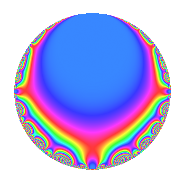# Properties

 Label 21.2.eLevel 21 Weight 2 Character orbit e Rep. character $$\chi_{21}(4,\cdot)$$ Character field $$\Q(\zeta_{3})$$ Dimension 2 Newforms 1 Sturm bound 5 Trace bound 0

# Learn more about

## Defining parameters

 Level: $$N$$ = $$21 = 3 \cdot 7$$ Weight: $$k$$ = $$2$$ Character orbit: $$[\chi]$$ = 21.e (of order $$3$$ and degree $$2$$) Character conductor: $$\operatorname{cond}(\chi)$$ = $$7$$ Character field: $$\Q(\zeta_{3})$$ Newforms: $$1$$ Sturm bound: $$5$$ Trace bound: $$0$$

## Dimensions

The following table gives the dimensions of various subspaces of $$M_{2}(21, [\chi])$$.

Total New Old
Modular forms 10 2 8
Cusp forms 2 2 0
Eisenstein series 8 0 8

## Trace form

 $$2q$$ $$\mathstrut -\mathstrut 2q^{2}$$ $$\mathstrut -\mathstrut q^{3}$$ $$\mathstrut -\mathstrut 2q^{4}$$ $$\mathstrut +\mathstrut 2q^{5}$$ $$\mathstrut +\mathstrut 4q^{6}$$ $$\mathstrut -\mathstrut 5q^{7}$$ $$\mathstrut -\mathstrut q^{9}$$ $$\mathstrut +\mathstrut O(q^{10})$$ $$2q$$ $$\mathstrut -\mathstrut 2q^{2}$$ $$\mathstrut -\mathstrut q^{3}$$ $$\mathstrut -\mathstrut 2q^{4}$$ $$\mathstrut +\mathstrut 2q^{5}$$ $$\mathstrut +\mathstrut 4q^{6}$$ $$\mathstrut -\mathstrut 5q^{7}$$ $$\mathstrut -\mathstrut q^{9}$$ $$\mathstrut +\mathstrut 4q^{10}$$ $$\mathstrut +\mathstrut 2q^{11}$$ $$\mathstrut -\mathstrut 2q^{12}$$ $$\mathstrut +\mathstrut 2q^{13}$$ $$\mathstrut +\mathstrut 2q^{14}$$ $$\mathstrut -\mathstrut 4q^{15}$$ $$\mathstrut +\mathstrut 4q^{16}$$ $$\mathstrut -\mathstrut 2q^{18}$$ $$\mathstrut -\mathstrut q^{19}$$ $$\mathstrut -\mathstrut 8q^{20}$$ $$\mathstrut +\mathstrut 4q^{21}$$ $$\mathstrut -\mathstrut 8q^{22}$$ $$\mathstrut +\mathstrut q^{25}$$ $$\mathstrut -\mathstrut 2q^{26}$$ $$\mathstrut +\mathstrut 2q^{27}$$ $$\mathstrut +\mathstrut 8q^{28}$$ $$\mathstrut +\mathstrut 8q^{29}$$ $$\mathstrut +\mathstrut 4q^{30}$$ $$\mathstrut -\mathstrut 9q^{31}$$ $$\mathstrut +\mathstrut 8q^{32}$$ $$\mathstrut +\mathstrut 2q^{33}$$ $$\mathstrut -\mathstrut 2q^{35}$$ $$\mathstrut +\mathstrut 4q^{36}$$ $$\mathstrut -\mathstrut 3q^{37}$$ $$\mathstrut -\mathstrut 2q^{38}$$ $$\mathstrut -\mathstrut q^{39}$$ $$\mathstrut -\mathstrut 20q^{41}$$ $$\mathstrut -\mathstrut 10q^{42}$$ $$\mathstrut +\mathstrut 10q^{43}$$ $$\mathstrut +\mathstrut 4q^{44}$$ $$\mathstrut +\mathstrut 2q^{45}$$ $$\mathstrut +\mathstrut 6q^{47}$$ $$\mathstrut -\mathstrut 8q^{48}$$ $$\mathstrut +\mathstrut 11q^{49}$$ $$\mathstrut -\mathstrut 4q^{50}$$ $$\mathstrut -\mathstrut 2q^{52}$$ $$\mathstrut -\mathstrut 12q^{53}$$ $$\mathstrut -\mathstrut 2q^{54}$$ $$\mathstrut +\mathstrut 8q^{55}$$ $$\mathstrut +\mathstrut 2q^{57}$$ $$\mathstrut -\mathstrut 8q^{58}$$ $$\mathstrut +\mathstrut 12q^{59}$$ $$\mathstrut +\mathstrut 4q^{60}$$ $$\mathstrut -\mathstrut 10q^{61}$$ $$\mathstrut +\mathstrut 36q^{62}$$ $$\mathstrut +\mathstrut q^{63}$$ $$\mathstrut -\mathstrut 16q^{64}$$ $$\mathstrut +\mathstrut 2q^{65}$$ $$\mathstrut +\mathstrut 4q^{66}$$ $$\mathstrut +\mathstrut 5q^{67}$$ $$\mathstrut -\mathstrut 16q^{70}$$ $$\mathstrut -\mathstrut 12q^{71}$$ $$\mathstrut +\mathstrut 3q^{73}$$ $$\mathstrut -\mathstrut 6q^{74}$$ $$\mathstrut +\mathstrut q^{75}$$ $$\mathstrut +\mathstrut 4q^{76}$$ $$\mathstrut -\mathstrut 8q^{77}$$ $$\mathstrut +\mathstrut 4q^{78}$$ $$\mathstrut +\mathstrut q^{79}$$ $$\mathstrut -\mathstrut 8q^{80}$$ $$\mathstrut -\mathstrut q^{81}$$ $$\mathstrut +\mathstrut 20q^{82}$$ $$\mathstrut +\mathstrut 12q^{83}$$ $$\mathstrut +\mathstrut 2q^{84}$$ $$\mathstrut -\mathstrut 10q^{86}$$ $$\mathstrut -\mathstrut 4q^{87}$$ $$\mathstrut -\mathstrut 16q^{89}$$ $$\mathstrut -\mathstrut 8q^{90}$$ $$\mathstrut -\mathstrut 5q^{91}$$ $$\mathstrut -\mathstrut 9q^{93}$$ $$\mathstrut +\mathstrut 12q^{94}$$ $$\mathstrut +\mathstrut 2q^{95}$$ $$\mathstrut +\mathstrut 8q^{96}$$ $$\mathstrut -\mathstrut 12q^{97}$$ $$\mathstrut +\mathstrut 4q^{98}$$ $$\mathstrut -\mathstrut 4q^{99}$$ $$\mathstrut +\mathstrut O(q^{100})$$

## Decomposition of $$S_{2}^{\mathrm{new}}(21, [\chi])$$ into irreducible Hecke orbits

Label Dim. $$A$$ Field CM Traces $q$-expansion
$$a_2$$ $$a_3$$ $$a_5$$ $$a_7$$
21.2.e.a $$2$$ $$0.168$$ $$\Q(\sqrt{-3})$$ None $$-2$$ $$-1$$ $$2$$ $$-5$$ $$q+(-2+2\zeta_{6})q^{2}-\zeta_{6}q^{3}-2\zeta_{6}q^{4}+\cdots$$